## Introduction

There are various forms of elevators since several hundred years. At first, they were crane-like mechanical lifts with drum-and- winch mechanisms powered by humans, draft animals, water wheels or steam. Block-and-tackle roping allowed even the heaviest loads to be lifted. For repeated use, inventers quickly learned to counterbalance the weight of the lifting platform or car cage in order to reduce the effort required to move expected payloads up and down the hoistway. Many improvements toward elevator safety and practical electric motors were invented near the end of the 19th century. Despite scientific disputes about AC versus DC electric power distribution, almost all elevators were converted and built to be powered by electric motors by the 1920s. Drum-and-winch operation gave way to counterweighted systems with direct traction sheaves on steel suspension ropes.

Despite many improvements toward safety and ease of use, the elevators of today still use the same counterweighted lifting mechanism and a traction sheave on the suspension ropes for the simple reason that counterbalancing the weight (mass) of the car against gravity reduces the amount of force required to move the car up and down the hoistway. Also, when additional counterweight is added to balance the system with an average payload, the least amount of effort is required for the expected range of typical loads. Less effort to move an average load also means less average energy consumption. In today’s world, energy conservation is one of the major goals for improvement. We typically pay for energy as measured by the kilowatt-hour utility meter. Whatever we can do to conserve and minimize energy use will certainly help save money and conserve energy supplies.

## Elevator design

The elevator design starts with making a first guess on required power, speed and gear ratio. An animation of the elevator drive visualises the movement of the cage and counterweight.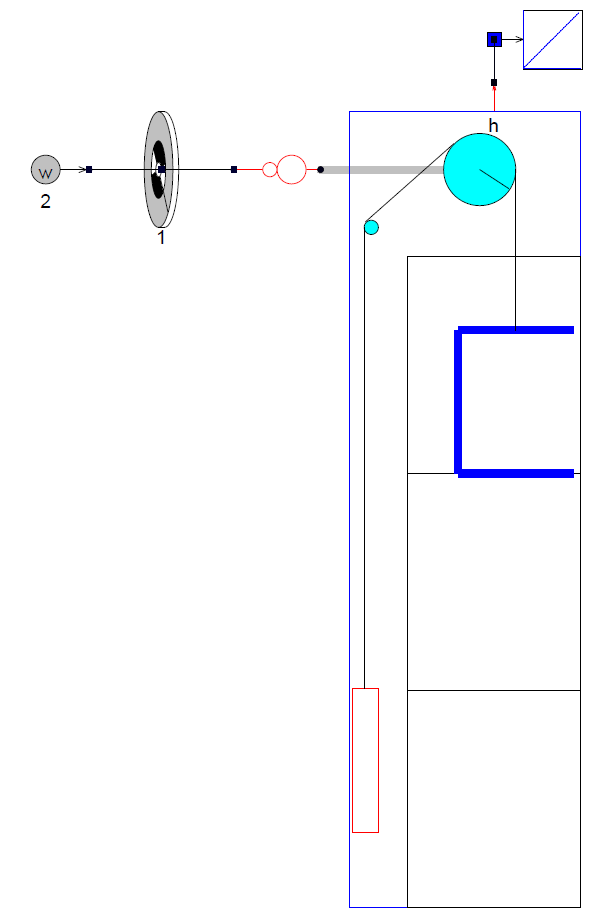Positioning the elevator requires a position control that can be either build arounda controlled torque or controlled speed drive.

## Constant speed elevator

To get a first understanding on the angular shaft speed movement of the elevator we are going to test the elevator with a constant angular speed. We want the elevator to move over a distance of $$h=6$$ meters during $$t=10$$ seconds. The radius of the wheel equals $$R=0.4$$ meter. From this the shaft angular speed can be calculated as $\theta = \frac{h}{R}=\frac{6}{0.4} = 15\\ \text{Constant Speed } v = \frac{\theta}{t}= \frac{15}{10}= 1.5 [Rad/s]$

The shaft angular speed $$\omega$$ is measured as well as the distance $$x$$ of the elevator.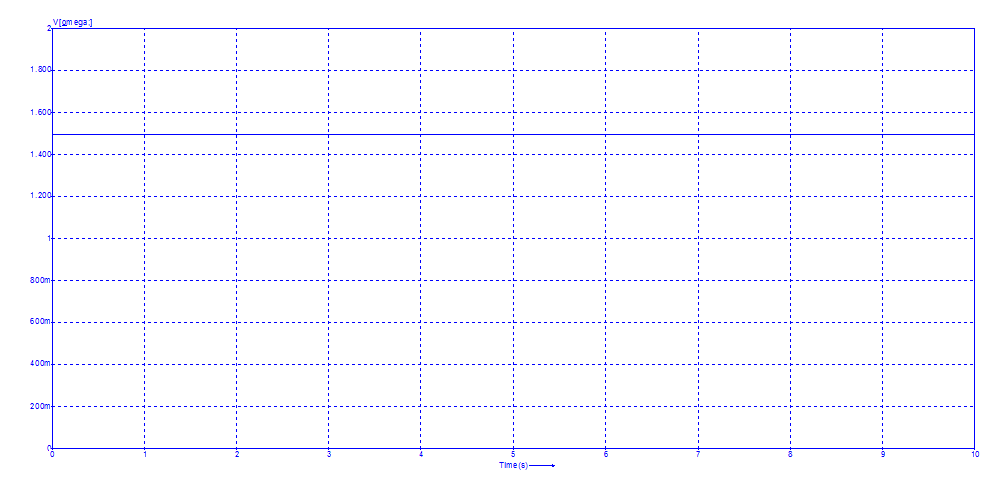Since the angular speed of the shaft is constant, the elevator moves with constant speed.

Since the accelerator $$d\omega \over dt$$ and Jerk $$d^2\omega \over dt^2$$ are too high in this case, position control is required to make the ride more smooth

## Mechanical model

The mechanical model of the elevator contains the wheel, gear box and masses of the cabin and counterweight. Using the R2T blocks the rotating shaft is coupled to the vertical movement of the cabin and counterweight. The efficiency of the drive components is modeled inside the gearboxes.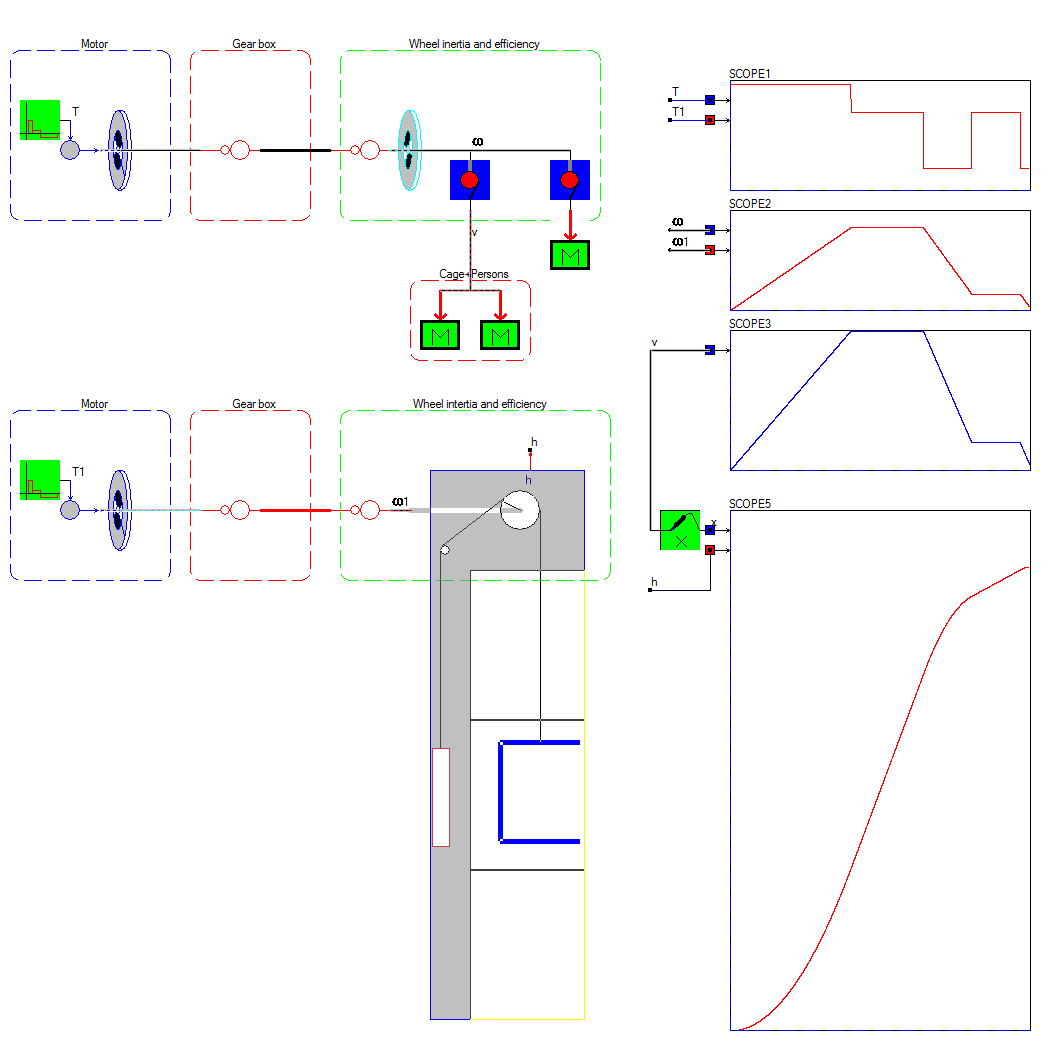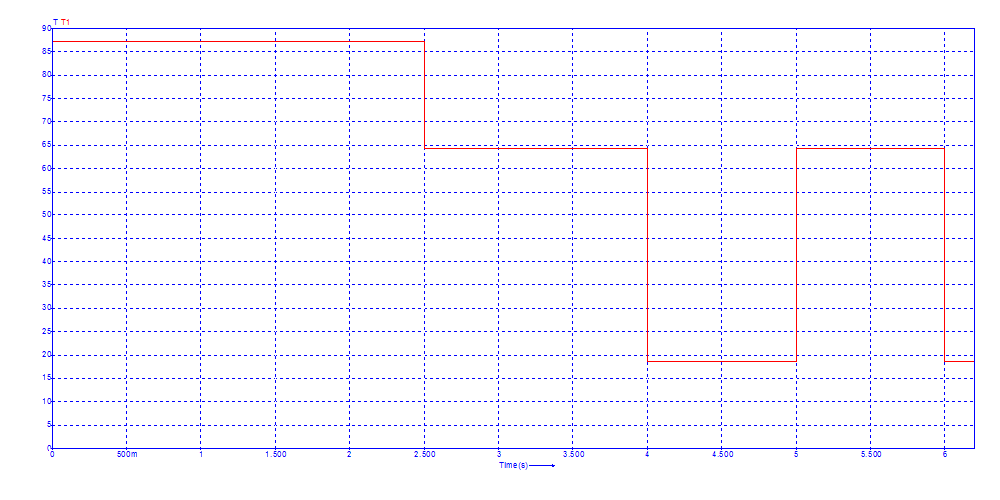Comparison between mechanical model and library model.

## Designing the torque profile

If the movement is known a priori, the driving torque profile can be defined to feed the drive shaft. However, using the block ProfilePosition from Components/Blocks/Sources, it all becomes a lot easier, as now we only have to define the required distance and the maximum speed, acceleration and jerk. For more info on the block ProfilePostion, see the tutorial on Variable Speed Drives VSD.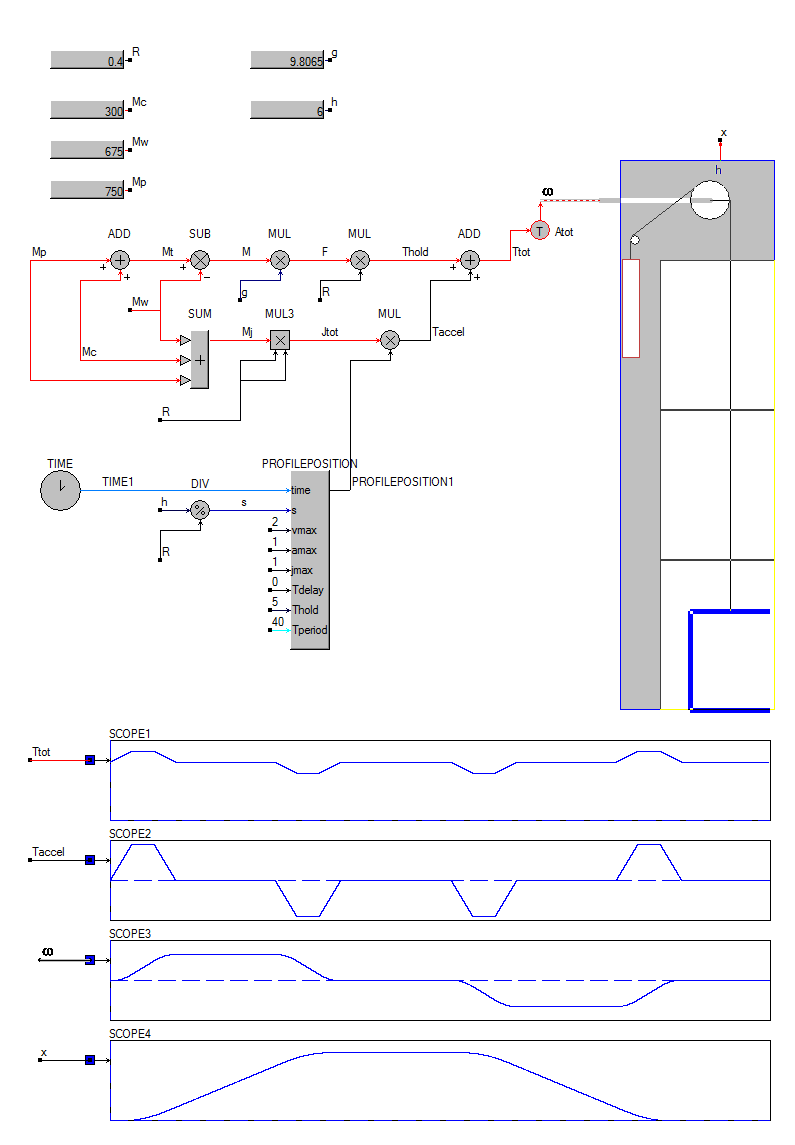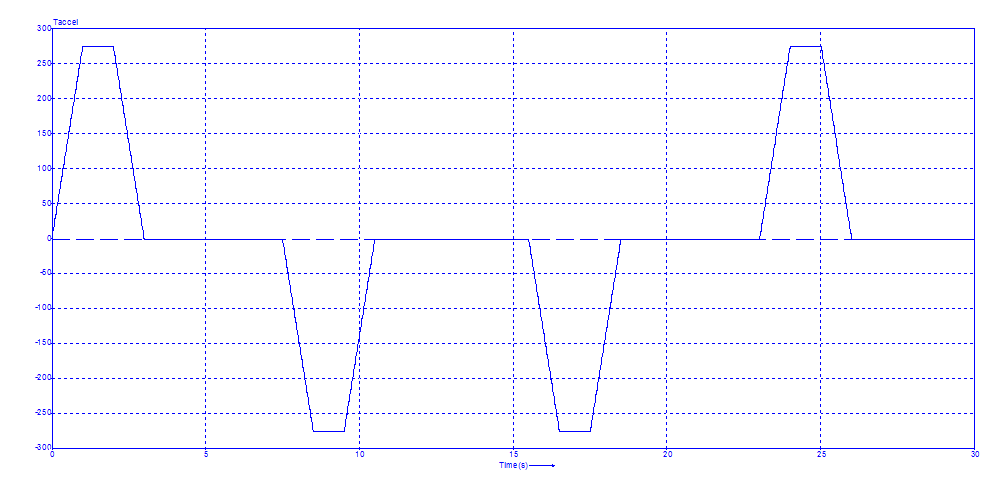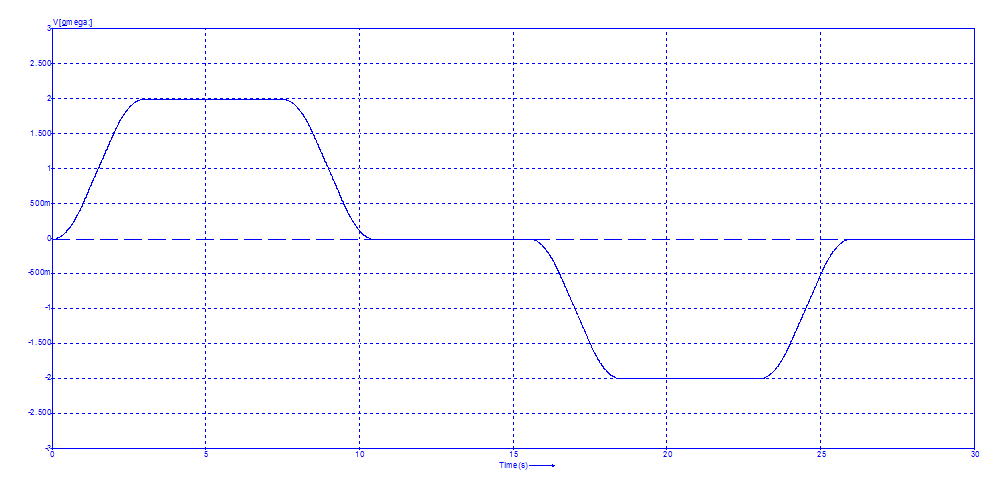Torque profile.

## Variable speed drive with torque profile

The torque profile can directly control a variable speed drive. For more info on the block ProfilePostion and variable speed drives, see the tutorial on Variable Speed Drives VSD.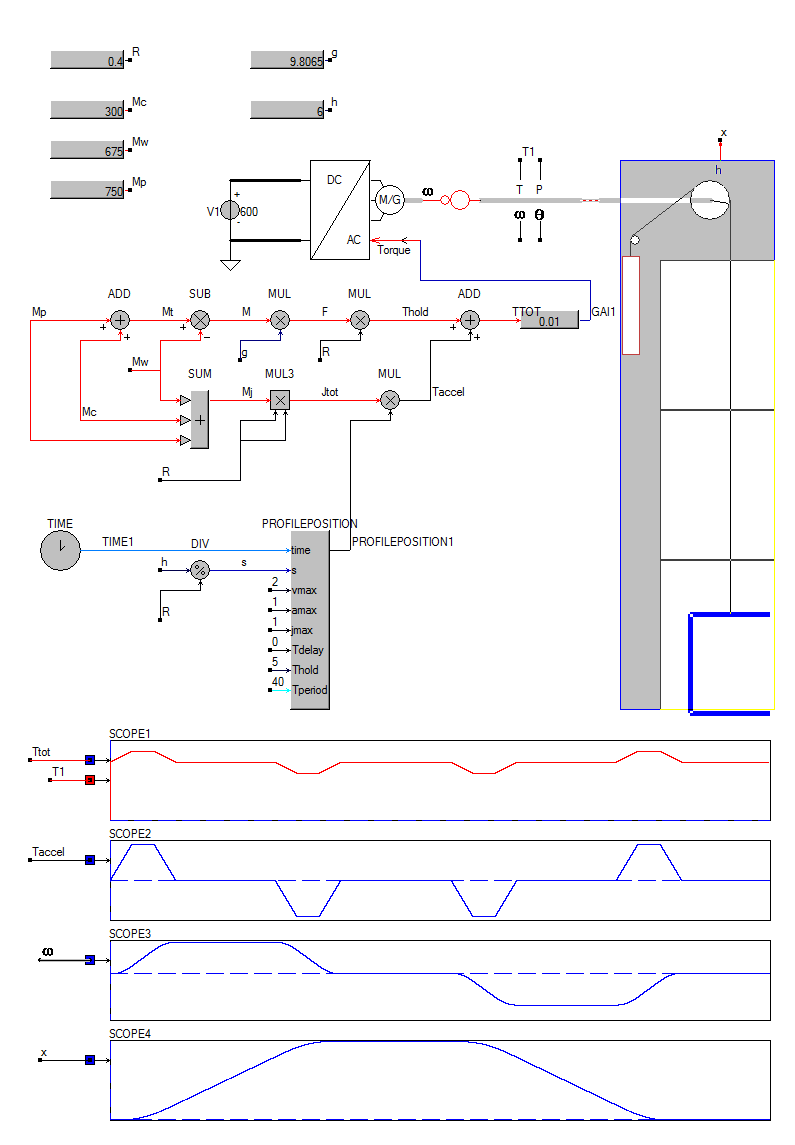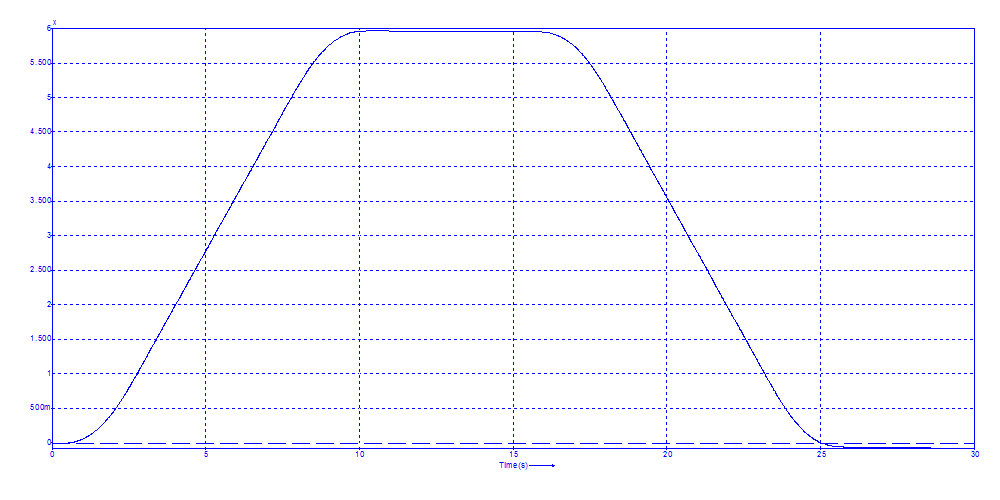Torque profile feeds a Variable Speed Drive.

## Speed controlled elevator VSD

The problem of regulating the amount of torque in the drive is that you have to know all parameters of the drive a priori. This is not possible, since you will never know the amount of persons or goods inside the cabin. A speed control will allow a movement for varying load. From the ProfilePosition block the required speed is calculated using an ideal integrator block. This speed signal is multiplied with 100 to account for the speed reduction of the gearbox.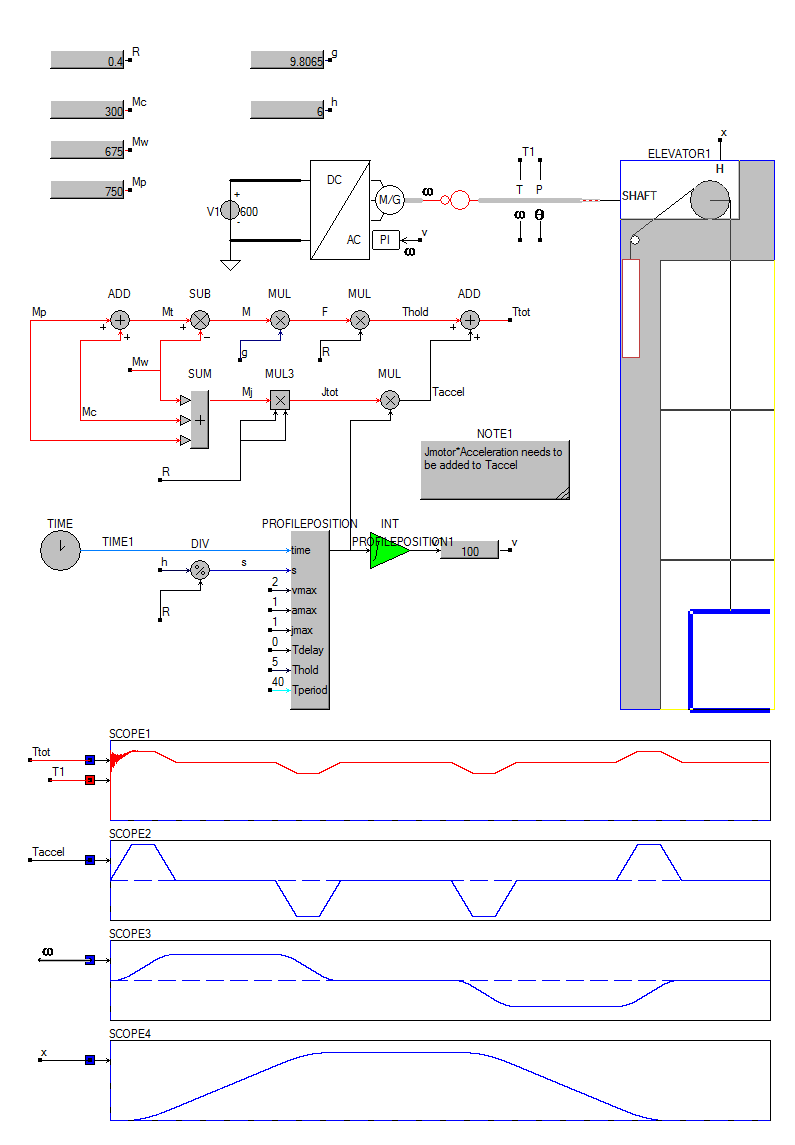The profile block calculates the required acceleration of the elevator and from this the required speed is calculated using a ideal integrator. This speed signal $$v$$ is input of the Variable Speed Drive block, which has an internal PI controller.

## Jerk in a speed controlled elevator

Jerk is calculated from the measured angular speed. Minimizing Jerk during starting and stopping the elevator increases comfort for the cabin passengers.

Acceleration: $\alpha = {d\omega \over dt }\\$ Jerk: $J ={ d^2\omega \over dt^2} = {d\alpha \over dt }$ The Jerk form the drive is more or less damped by the elasticity of the rope.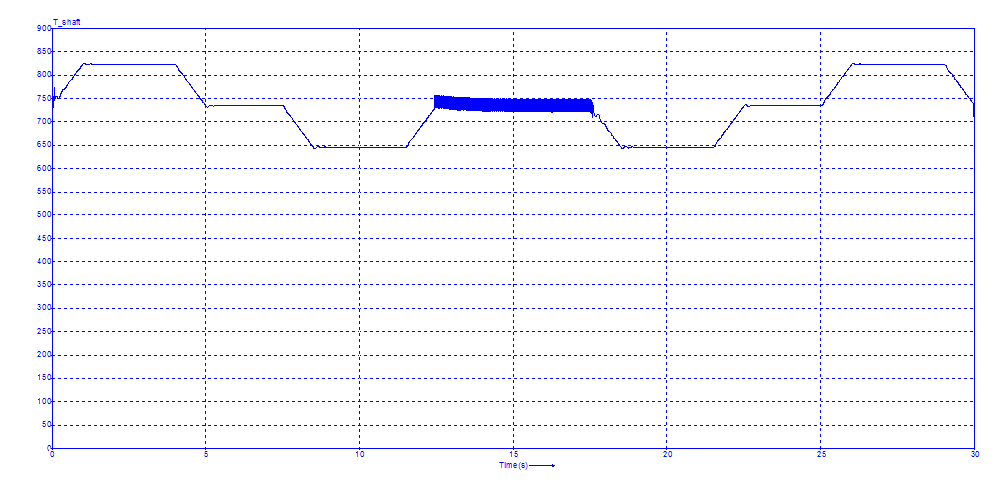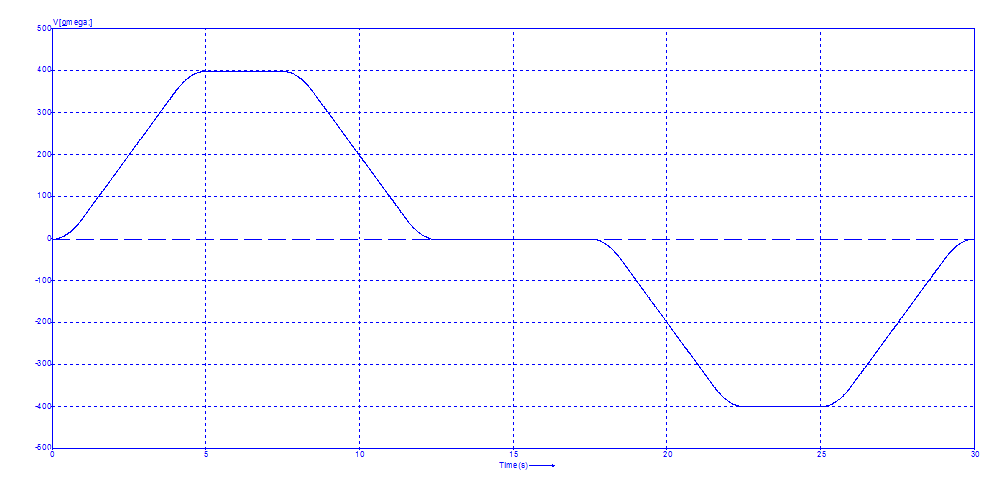Jerk is calculated from the measured angular speed, by differentiating it twice using and ideal differentiator.

## Adding a brake for standstil operation

To remove Jerk during standstill, a mechanical brake is added which comes into action when the reference angular speed equals zero $$\omega=0$$.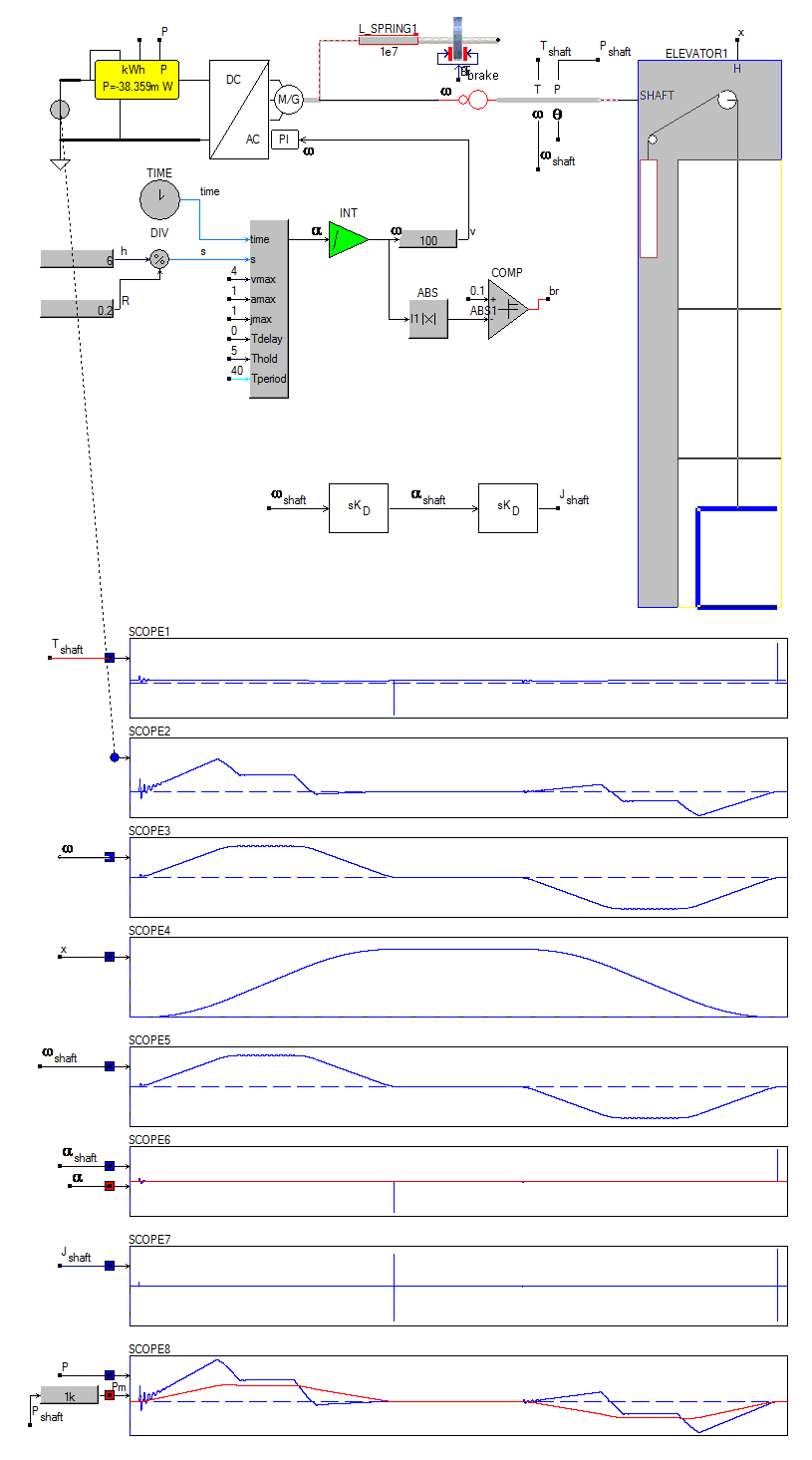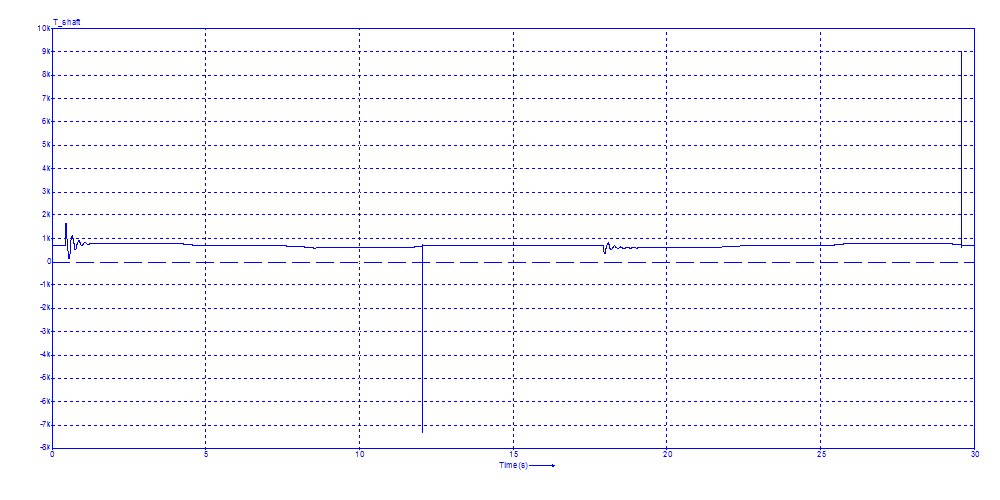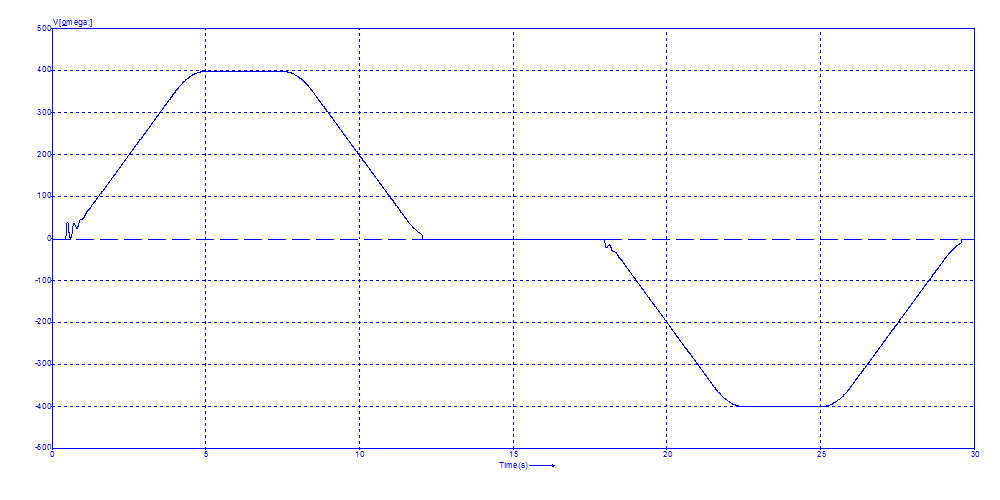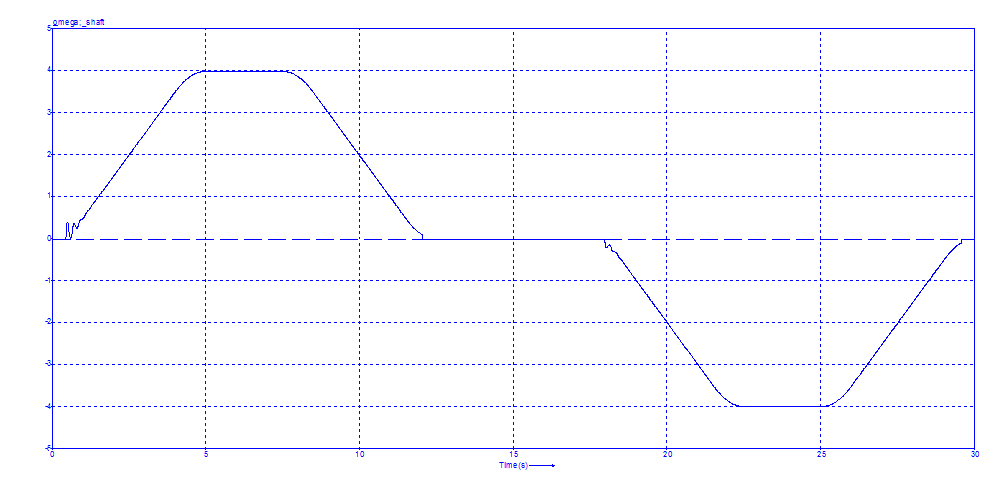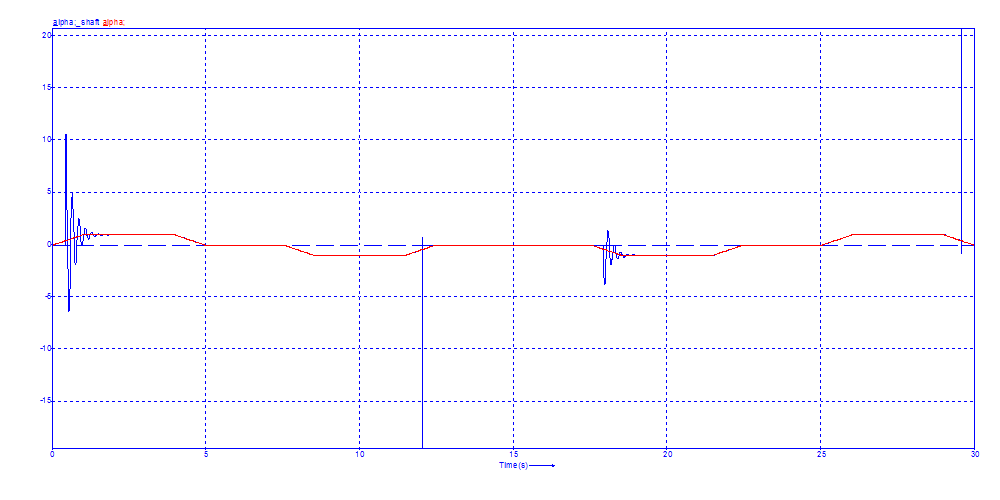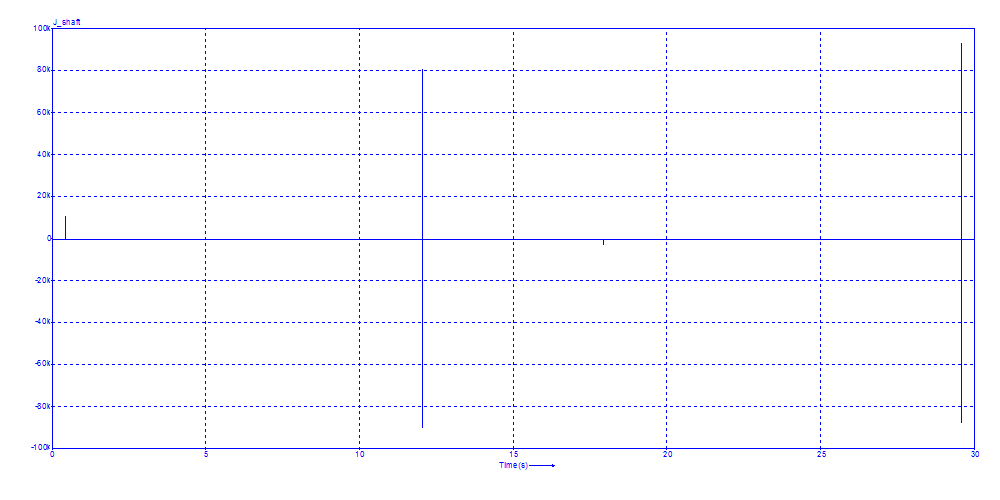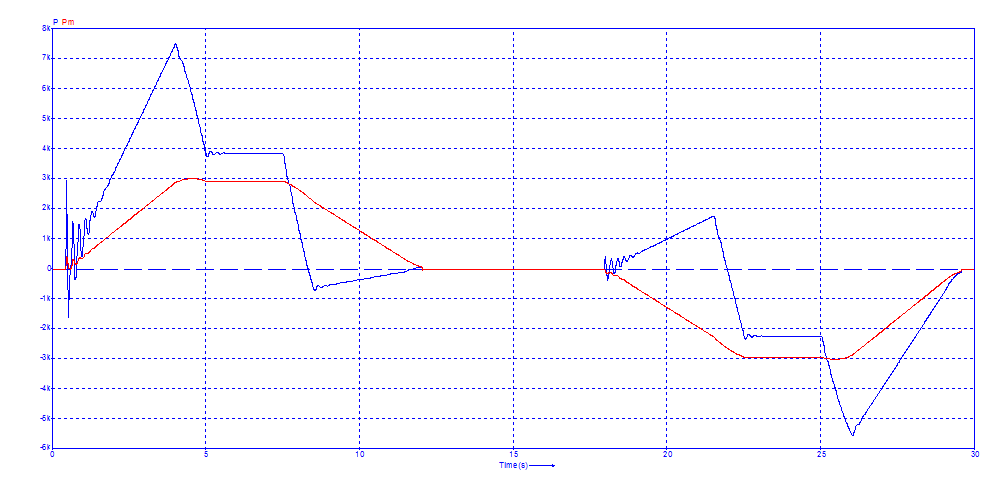The brake is activated when ω is below 0.1 Rad/s.